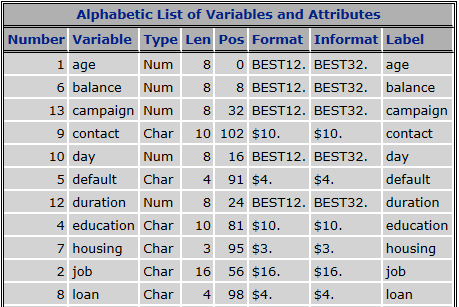## proc content out put position

Can any body help me out what is POS in the proc content out put and how system define the position the variable1 ACCEPTED SOLUTION

Accepted Solutions

## Re: proc content out put position

@mrinmoy wrote:

Are you telling that in proc content output the variable order is showing alphabaticaly but machine read first numarical variable then it reads char var.

This is how SAS stores variables within a dataset observation.

First come 8-byte and 4-byte numeric variables. This is done so that most numeric variables align with 4- or 8-byte boundaries, which makes moving them in memory more efficient.

Then come the other numeric variables (length = 3,5,6,7), then the strings.

SAS keeps a logical sequence of variables (the VARNUM), which corresponds to the sequence in which variables appeared when the dataset was created. This is either the sequence in which the datastep compiler encountered the variables when compiling the code and setting up the PDV, or the sequence of variables in a select in proc sql. This sequence is used for displaying, proc print etc.

7 REPLIES 7

## Re: proc content out put position

POS is the starting position of the variable within the record (file observation).

Mainly you need no to care about it and it may change with data manipulation.

## Re: proc content out put position

Could you please explain me how sas is defining the variable position. For reff kindly go through the attached doc.## Re: proc content out put position

Hi.

It's the physical position inside the dataset file.

If you want to change this, one easy way is to recreate the table with PROC SQL:

proc sql noprint;

create table TAB as

select COL1, COL2, COL3, ... from TAB;

quit;

Hope it helps.

Daniel Santos @ www.cgd.pt

## Re: proc content out put position

- 1st variable is AGE starting POS=0 with length=8

- 2nd variable need start at pos=8 (=0+8)  is balance with length=8.

- 3rd variable need start at pos=16 (=8+8) is day with length=8.

- 4th variable need start at pos=24 (=16+8) is duration with length=8

As you can see, all numeric variables are length 8 and appear at the beginning of the record.

The 1st character variable is job starting pos=56 with length=16,

so next char variable need start at pos=72 (=56+16) ...etc

## Re: proc content out put position

Are you telling that in proc content output the variable order is showing alphabaticaly but machine read first numarical variable then it reads char var.

## Re: proc content out put position

Hi.

Could it be that what you are looking for is the ORDER= option?

Hope it helps.

Daniel Santos @ www.cgd.pt

## Re: proc content out put position

@mrinmoy wrote:

Are you telling that in proc content output the variable order is showing alphabaticaly but machine read first numarical variable then it reads char var.

This is how SAS stores variables within a dataset observation.

First come 8-byte and 4-byte numeric variables. This is done so that most numeric variables align with 4- or 8-byte boundaries, which makes moving them in memory more efficient.

Then come the other numeric variables (length = 3,5,6,7), then the strings.

SAS keeps a logical sequence of variables (the VARNUM), which corresponds to the sequence in which variables appeared when the dataset was created. This is either the sequence in which the datastep compiler encountered the variables when compiling the code and setting up the PDV, or the sequence of variables in a select in proc sql. This sequence is used for displaying, proc print etc.

Discussion stats
• 7 replies
• 1410 views
• 3 likes
• 4 in conversation